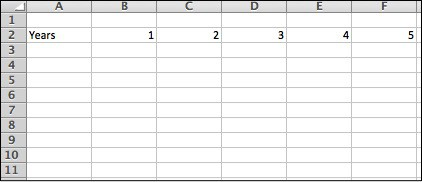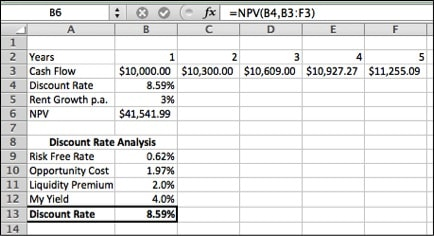# Discounted Cash Flow Analysis 101Ankit Duggal

## As a Guest you have free article(s) left

As Ali Boone’s article titled Rental Property Numbers so Easy that You Can Calculate Them on Napkin pointed out, “In this industry, you must love the numbers. Love them like they are part of you. For good or for bad, ‘til death do you part, never leave the numbers.”

Knowing how to quickly analyze deals to ascertain their cash flow is the key skill set needed by an income oriented real estate investor. My goal with this article is to build on top of the great foundation laid by Ali to show you to how build out a Discount Cash Flow Analysis Model.

## What is a Discounted Cash Flow Model?

A discounted cash flow (DCF) analysis is a method of valuing an asset using the concepts of the time value of money. All future cash flows are estimated and discounted to give their present value   (PVs) — the sum of all future cash flows, both incoming and outgoing, is the net present value (NPV), which is taken as the value or price of the cash flows in question. Simplifying that cumbersome definition is that DCF analysis helps determine the value of an item/asset/company based on discounting back to today the cash flow that this asset can produce during your target holding period.

## What You Need to Build a DCF Model?

You will need two things to build your very own DCF model:

1. You will need access to a spreadsheet program. I utilize Microsoft Excel but if you do not want to pay for that program then you get a great proxy of that program at Open Office or through Google Docs.
2. You are going to need data on the items that Ali wrote about in her past article relating to the income and the expense categories. So have those number handy and close by.

### How to Build your Own DCF Model?

Now that you have the two items needed to build your model. Lets start building this model from scratch:

Step I- Open a New Spreadsheet Document

Tip: Name and Save the spreadsheet upfront as the last thing you want to do is loose your work incase your close out or power goes from your laptop. It has happened to me so learn from my lessons.

Step II- There are two components to a DCF calculation: The Cash Flows and the Discount Rate.

Cash Flows: In our case will be net cash remaining after all expenses associated with the property are paid off so basically any cash flow that you as the equity investor will receive.

Discount Rate: The required rate of return that you demand on your money. Remember: we advised you to include this rate of return in your investment plan as well. It will all come together. So time to take that number of the out of the plan and input into your DCF model!

These two components when used together will help determine the Present Value (PV) of your next investment asset.

Open the blank spreadsheet and enter “years” in Cell A2 and the numbers 1-5 in cells B2:F2 to represent the year numbers for our worksheet as per the example below:Next we are going to enter the first year Net Operating Income (NOI)

Assumption: The first year net cash flow is \$10,000; Enter that amount in cell B3.

The next part of a DCF Analysis is to project the growth of income and expense over the horizon of your holding period. For the sake of simplicity we will be using just the NOI t is normal to use 3% for income growth rate year over year but make sure that the growth rate is feasible within your rental market. Enter 3% in cell B5 and lets grow our cash flow by 3% each year. Enter the following formula

=B3*(1+\$B\$5) into Cell C3 to grow the cash flow by 3% from the prior year and then click the outside right part of the cell and drag it across the columns until F3.Next we will put the labels Cash Flow and Discount Factor in cells A3:A4 respectively as per the example below:Next, in cell B5 input the rate at which you wish to discount the future cash flow back to today’s dollars.

Discount Rate percentage can simply be what you want to make per annum or you can use the following formula as guide post in helping you ascertain the target risk-free rate:

Risk Free Rate (10 Year Treasury Rate)

+ Opportunity Cost (5 Year CD Rate or S&P500 Dividend Yield)

+ Liquidity Premium (Real Estate is a illiquid asset so charge a premium for the risk)

+ Investor Return Percentage (What is the annual return that you wish to make)

= Your Personalized Discount Rate Percentage

**My discount rate analysis is indicated in the excel snapshot below:Next we need to calculate what is the Net Value of the asset’s future discounted cash flows. For that you use a formula called Net Present Value (NPV).

Net Present Value compares the value of a dollar today to the value of that same dollar in the future, taking inflation and returns into account. If the NPV of a prospective project is positive, it should be accepted.

You can use the formula below to both discount the cash flow and calculate the NPV value of your asset:

=NPV(B4, B3;F3)

Lastly the enter the heading NPV into cell A6, then go to cell B6 and enter the formula shown above.

So was your NPV positive or negative?In our example above the NPV was positive which means that the project should be accepted as it will produce positive cash flows based on your personalized discount rate.  In addition, this analysis indicates that the value of \$53,092 of future cash flow is \$41,542 today. That is time value of money folks as a dollar tomorrow is worth less than a dollar today!

Disclaimer: This is a very basic DCF model that ignores capital repairs, income changes, expense changes. I wanted to put this post together to just help new investors get a good grab on what a DCF model is all about and how they can use the tool to make income investment decision.

Build your very own DCF model and analyze your future deals with ease.

Happy Investing 🙂
Lauren Manning